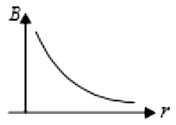A neutron of mass m enters a uniform magnetic field B with the velocity v perpendicular to it. The radius of the circular path made by a neutron is

1.

2.

3.

4.  $\mathrm{Zero}$

Complete Question Bank + Test Series
Complete Question Bank

Difficulty Level:

Two similar coils of radius R are lying concentrically with their planes at right angles to each other. The current flowing in them are 3I and 4I respectively. The resultant magnetic field induction at the center is

1.

2.

3.

4.   $\mathrm{Zero}$

Complete Question Bank + Test Series
Complete Question Bank

Difficulty Level: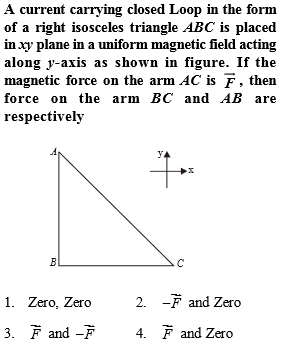Complete Question Bank + Test Series
Complete Question Bank

Difficulty Level:

A uniform electric field and a uniform magnetic field are acting in the same direction in a certain region. If a proton is projected in this region such that its velocity is pointed along direction of fields, then the proton

1.  will turn towards left of the direction of motion

2.  will turn towards the right of the direction of motion

3.  will speed up

4.  will slow down

Complete Question Bank + Test Series
Complete Question Bank

Difficulty Level:

When a proton is released from rest in a room, it stars with an initial acceleration ${\mathrm{a}}_{0}$ towards east. When it is projected towards north with a speed ${\mathrm{v}}_{0}$, it moves with initial acceleration $3{\mathrm{a}}_{0}$ towards east. The electric and magnetic fields in the room are

1.

2.

3.

4.

Complete Question Bank + Test Series
Complete Question Bank

Difficulty Level:

1.  In a circle

2.  In y-z plane

3.  With constant speed

4.  All of these are correct

Complete Question Bank + Test Series
Complete Question Bank

Difficulty Level:

If the planes of two identical concentric coils are the perpendicular and magnetic moment of each coil is M, then the resultant magnetic moment of the two coil system is

1.  M

2.  $\sqrt{2}\mathrm{M}$

3.  3M

4.  2M

Complete Question Bank + Test Series
Complete Question Bank

Difficulty Level:

A voltage has a resistance G and it can measure a maximum voltage of V. To convert it in a voltmeter of range nV, the volume of resistance required is

1.  (n - 1)G in series

2.  (n - 1)G in parallel

3.   in parallel

4.   in series

Complete Question Bank + Test Series
Complete Question Bank

Difficulty Level:

A current wire is hidden in a wall. Its position can be located with the help of a

1.  Moving coil galvanometer

2.  Voltmeter

3.  hot wire ammeter

4.  Magnetic needle

Complete Question Bank + Test Series
Complete Question Bank

Difficulty Level:

which of the following graph correctly represents the variation of magnetic field induction with distance due to a thin wire carrying current.

1.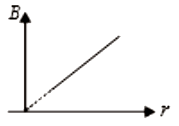2.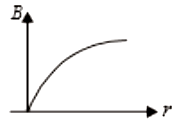3.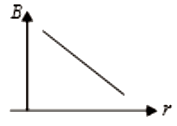4.